Start typing, then use the up and down arrows to select an option from the list.Jules Bruno
203views
12
so an example? One, it says calculate the pH off a 0.782 Moeller solution off. Calcium hydride. No, we know that calcium hydride is a strong base. Because remember, we have calcium, which is C A two plus connecting with h minus are hydride ions. So what happens here? The two from here came here. The one from here came here. That's how we got calcium hydride. And what we should realize here is that there are two calcium hydride in the formula. Now, this is what you need to remember. When it comes to strong basis for strong bases. You have to be very careful. We're going to say that this is not the real concentration of our strong base. You're gonna say when you have strong bases, you have to look at the number off O H minus H minus and H two minus 02 minus. So you have to look to see how many of each you have in order to determine the rial concentration off that strong base. In this particular question, we have to h minus is and because we have two of them, we have to multiply this by two that will give us the correct concentration off our strong base. Okay, so you have to remember that. So, for example, if I had C a o. H. Two and there was a 0.100 Moeller of it again because you have 20 H is you'd have to multiply that by two. If you have calcium of mind, you would have to an h two. So again, you have to multiply the concentration times to to get the correct concentration. Now, what we're gonna do is we're gonna multiply the initial concentration times to to get the rial concentration. Okay, When we do that, we're gonna get our correct concentration. That's the number that we're gonna plug in. So when we do that, and I'm gonna remove myself guys from the image so we have more room to work with When we do that, we're gonna get a new number a new value for r O. H. Minus concentration. So here, we're gonna get 0.1564 Mueller, that becomes our new concentration. So we're going to say p o. H equals negative. Log off O H minus. So it becomes negative. Log off 0.1564 Moeller. Now, some of you may be asking, but Jules, this is saying the concentration of O. H minus. We don't have O. H minus. We have h minus. How do they relate? Just realized that all four of these ions can be treated as being the same. They all feed into the concentration of O H minus. Okay, so h minus. Just understood as a general term for the strong basic ion off a strong base. So if I have to h 20 h minus is that's equivalent to two h minus is equivalent to two NH two minuses. Same thing. So when we take the negative log of this, this will give us our p o h. It's gonna give us 0.81 for our p o h. But remember, let's pay attention. I didn't ask for the pH I asked for. I didn't ask for the p o. H. I asked for the pH. So we need to use the next equation. PH plus p o h equals 14. Plug in what we know. For P o h. 0.81 we need ph. So subtract 0.81 from both sides. So ph here equals 13.19 So that will be our pH for this first question. Hopefully, guys didn't get lost. But what I was saying Just remember for strong bases you have to look to see how Maney o H minus is You have h minus is you have an H two minuses. You have an 02 businesses you have their number, determines the correct concentration of our strong base. And right now, what you need to realize is we only do this for strong base. At this point. If we had a strong acid such as H two s, 04 that strong acid has too acidic. H is. But we wouldn't need to multiply the concentration times too, because when it comes to acids, the first age coming off extremely strong. But the second one that comes off is extremely weak. And because the second H plus is extremely weak, we wouldn't need to multiply the concentration times too. So we only do this multiplication of concentrations when we have strong basis. Later on, when we deal with acid based titrate, shins and buffers, we'll talk about how assets play a role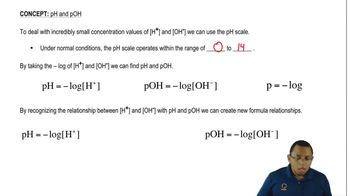01:31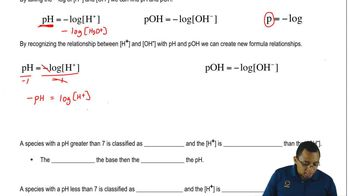01:35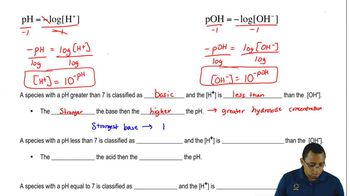02:42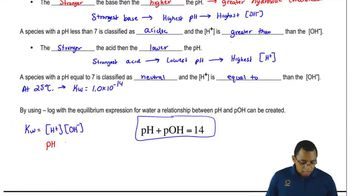01:21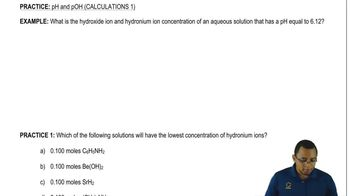03:15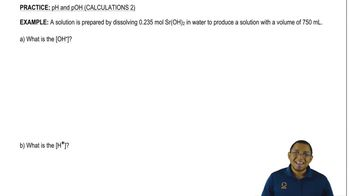04:07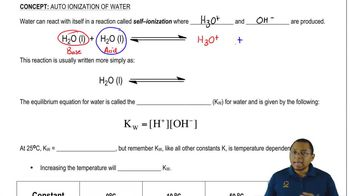03:10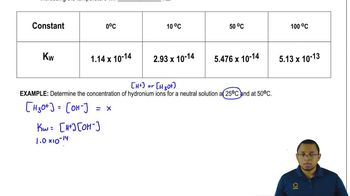02:47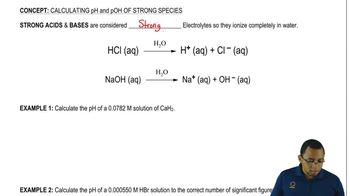01:32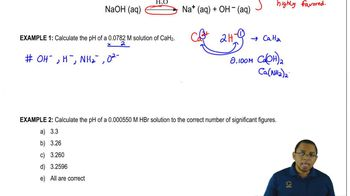04:48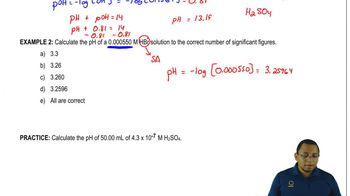03:29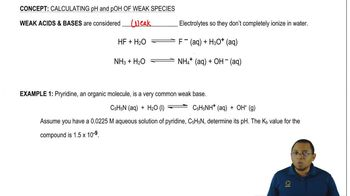00:57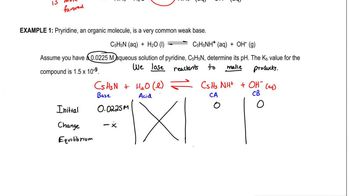09:40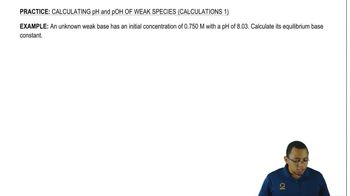05:31